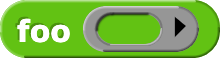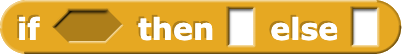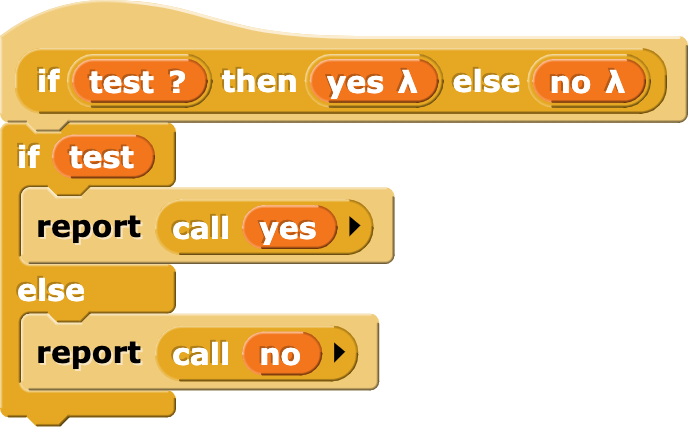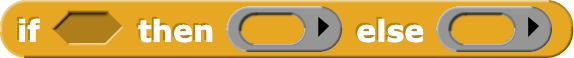# What does "(Unevaluated)" in an input type name mean?

Ordinarily, like most but not all programming languages, Snap! evaluates the inputs to a procedure before calling the procedure itself. That is, when you sayprocedure FOO knows that the value of its input is 5, but it doesn't know how that input was computed. It doesn't know that there's an addition involved; as far as FOO is concerned, it would make no difference if the expression wereThis evaluation rule (evaluate inputs first) is called "applicative order evaluation."

But Snap! allows exceptions to this rule. A procedure can say that it wants to get the expression for an input rather than that input's value when called.

In Snap!, how do we make an expression available as data? Right, we put a ring around it. The usual way to do that is to declare the input to be of type Reporter, so we have(This is generally more useful if the input expression has an empty slot, so that it can be re-evaluated with a different input each time, e.g.,

.)

Again, to get this behavior, you declare the input to be of type Reporter. That includes a ring in the block, into which you can drag an expression; you don't have to explicitly ringify the expression.

But sometimes you want to build a control structure that doesn't rub the user's nose in the fact that there's a procedure involved. The classic example isThis is now a primitive, but originally we wrote it in Snap!. Here was our first effort:Why do the YES and NO inputs have to be ringed?

Think about writing a recursive function, such as factorial:

So now we step through the computation of (FACTORIAL 3) supposing that YES and NO are ordinary inputs, computed before FACTORIAL is called. The IF reporter looks like this:

The THEN input expression is just 1, and its value is 1. The ELSE input expression, though, is (N × (FACTORIAL (N − 1))). Since N=3, the value of this expression is (3 × (FACTORIAL 2)). So before we can finish computing this input, we have to evaluate (FACTORIAL 2).

So, the THEN input is 1, and the ELSE input is (N × (FACTORIAL (N − 1))). So we have to compute (FACTORIAL 1).

This time, the THEN input is 1, and the ELSE input is (N × (FACTORIAL (N − 1))). So we have to compute (FACTORIAL 0).

This time, the THEN input is 1, and the ELSE input is (N × (FACTORIAL (N − 1))). So we have to compute (FACTORIAL -1).

This will go on forever, computing (FACTORIAL -2), which requires (FACTORIAL -3), and so on.

The reason this doesn't actually happen is that the THEN and ELSE inputs are ringed, and only one of them will actually be evaluated by CALL.

If YES and NO are declared as type Reporter, the block ends up looking like this:We can testify that students find this confusing. Also, you can't type a 1 into a ring. For both those reasons, we want the input slots to look like ordinary (evaluated) inputs, but behave like ringed (unevaluated) inputs. This is what "Any (unevaluated)" means.